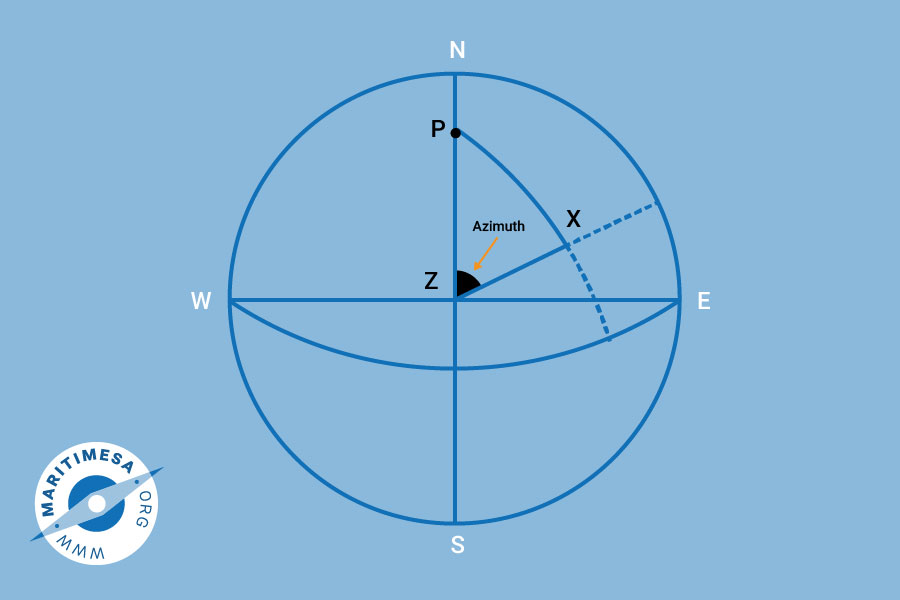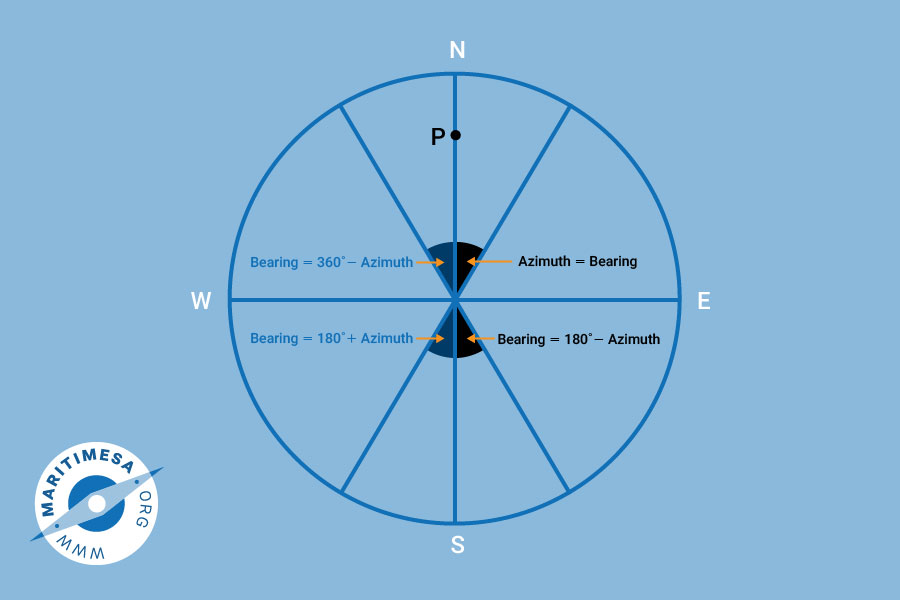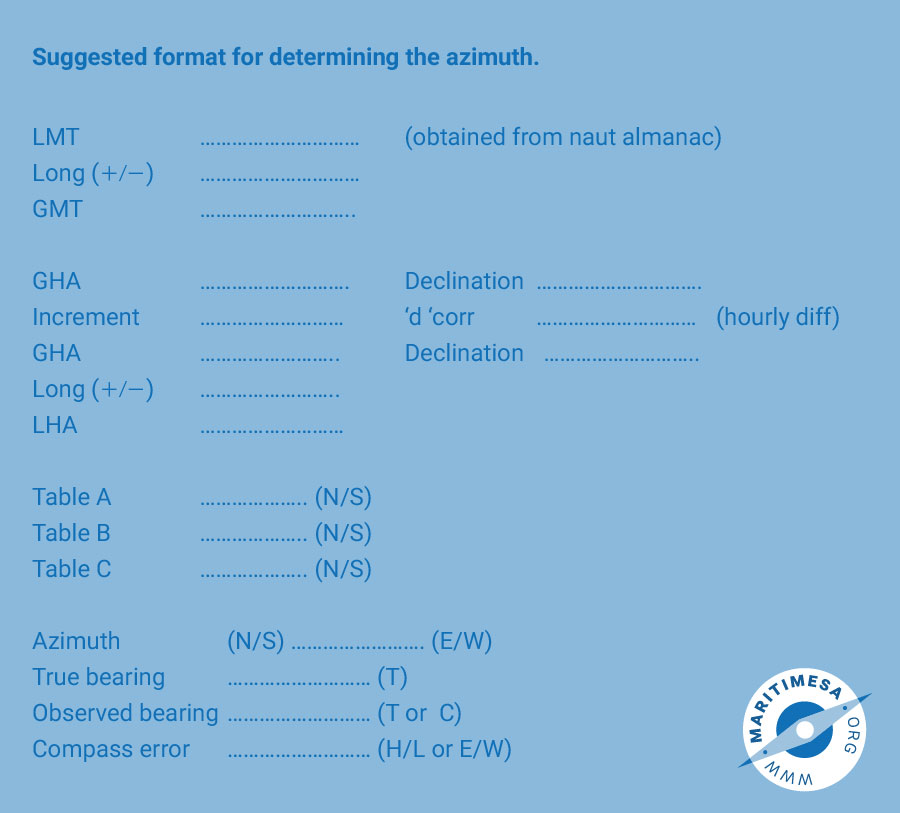# The azimuth

In order to determine the error of the gyro or magnetic compass by astronomical observation, one must be able to find the true bearing of a celestial body from the observer at any time. By comparing the compass bearing with the bearing calculated at a specific time, one can determine the error of the compass. An azimuth can be defined as the angle measured at the zenith between the direction of the elevated pole and the vertical circle passing through the body. It is measured from 0° to 180° either side of the observer’s meridian. It is named the same as the latitude and east if the body is rising and west if the body is setting. It is the angle Z in the PZX triangle and in order to find the azimuth, the triangle needs to be solved.Conversion of the azimuth to a true bearing.

1. If the azimuth is named north and east, then the bearing will be the same as the azimuth.
2. If the azimuth is named north and west, then the bearing will be 360° – azimuth.
3. If the azimuth is named south and east, then the bearing will be 180° – azimuth.
4. If the azimuth is namedsouth and west, then the bearing will be 180° + azimuth.Solution of azimuth

In order to determine the azimuth, use is made of the A,B. C tables in Nories. To enter the tables you require the following:

1. The Local Hour Angle.
2. The declination.
3. The DR latitude.

Table A is entered with the hour angle and the DR lat. The figure obtained is always named the opposite to the latitude when the hour angle is between 90° and 270°.

Table B is entered with hour angle and declination. The figure obtained is always named the same as the declination.

In order to enter table C we must first either add the results of table A and B or subtract one from the other. If they have the same names (ie both N or both S) then they are added . If they have different names, they are subtracted. C is named after the larger of A and B.

Table C is entered with C and the DR latitude. The figure obtained is named the same as the larger of A and B. The azimuth which is obtained takes the combined names of C and the hour angle.Notes:

1. If you are checking your compass for gyro error then the result will be categorised “high” or “low”. If you are checking your magnetic compass the result will be categorised as “east” or “west”.
2. If the true bearing is greater than the compass bearing, then the compass error is named E (east). If it is less than the compass bearing, the compass error is named W (west).